# FSC Mathematics MCQs Chapter 10 Class 11

NUST entry test 2023 NET contains most of the Mathematics MCQs. FSC Part 1 Mathbook MCQs. HSSC Math questions MCQs. Solved Chapterwise Math MCQs for first-year 11 class MCQs. FSC Math book Second-year and first-year fsc textbooks chapter-wise MCQs with the answer are provided here. PakLearningSpot PLS MCQs Bank website for FREE Entry test preparations

423
Created on By Ali Durrani

Math Ch 10 Trigonometric Identities

1 / 19

𝒔𝒊𝒏(𝟏𝟖𝟎° + 𝜶)𝒔𝒊𝒏(𝟗𝟎° − 𝜶) =

2 / 19

𝒔𝒆𝒄(−𝟑𝟎𝟎°) =

3 / 19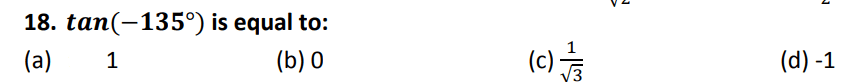4 / 19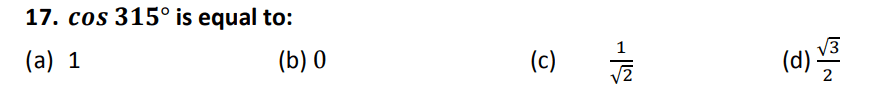5 / 196 / 19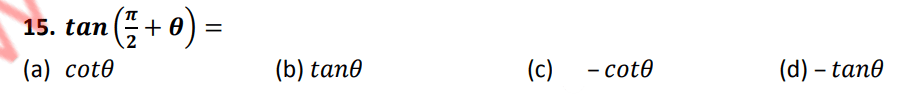7 / 19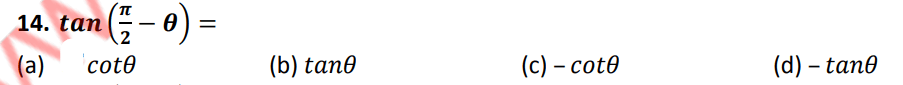8 / 19

Angles associated with basic angles of measure 𝜽 to a right angle or its multiple are
called:

9 / 19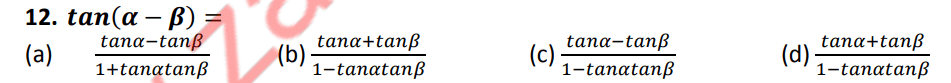10 / 1911 / 19

𝒔𝒊𝒏(𝟐𝝅 − 𝜽) =

12 / 19

𝒄𝒐𝒔(𝟐𝝅 − 𝜽) =

13 / 19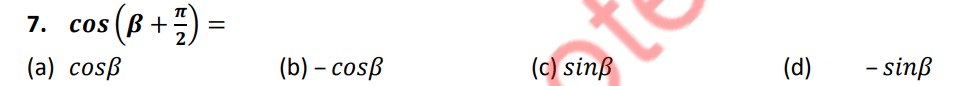14 / 19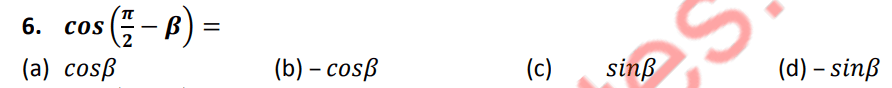15 / 19

𝒔𝒊𝒏(𝜶 − 𝜷) is equal to:

16 / 19

𝒔𝒊𝒏(𝜶 + 𝜷) is equal to:

17 / 19

𝒄𝒐𝒔(𝜶 + 𝜷) is equal to:

18 / 19

Fundamental law of trigonometry is , 𝒄𝒐𝒔(𝜶 − 𝜷)

19 / 19

Distance between the points 𝑨(𝟑, 𝟖) & 𝐵(5,6) is:

The average score is 62%

0%

### 2 responses to “FSC Mathematics MCQs Chapter 10 Class 11”

1. Arooj says:

In this McQ 4 option c is right

Salam